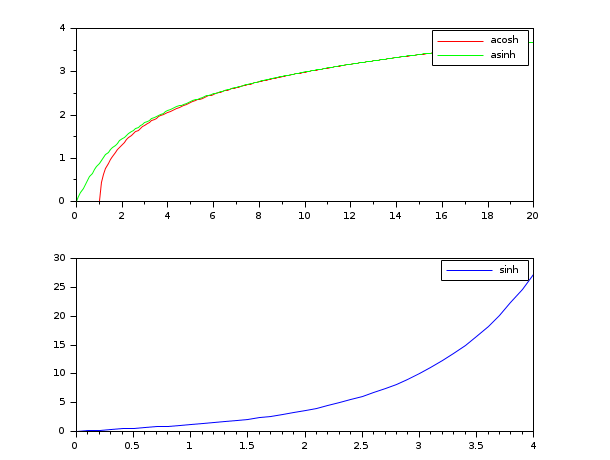# sca

set the current axes entity

### Syntax

`a=sca(a)`

### Arguments

a

a handle, the handle on the Axes entity

### Description

`sca(a)` is used to set the current `Axes` entity (see graphics_entities) to the one pointed to by the handle `a`. The drawing functions generally use the current axes entity.

### Examples

```clf()
a1=newaxes();
a1.axes_bounds=[0,0,1.0,0.5];
t=0:0.1:20;
plot(t,acosh(t),'r')
a2=newaxes();
a2.axes_bounds=[0,0.5,1.0,0.5];
x=0:0.1:4;
plot(x,sinh(x))

sca(a1); //make first axes current
plot(t,asinh(t),'g')
legend(['acosh','asinh'])
sca(a2); //make second axes current
legend('sinh')```